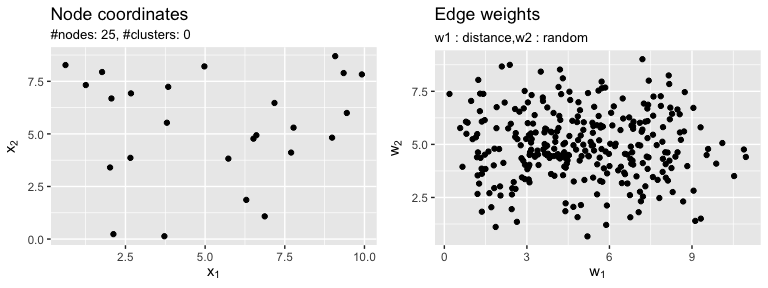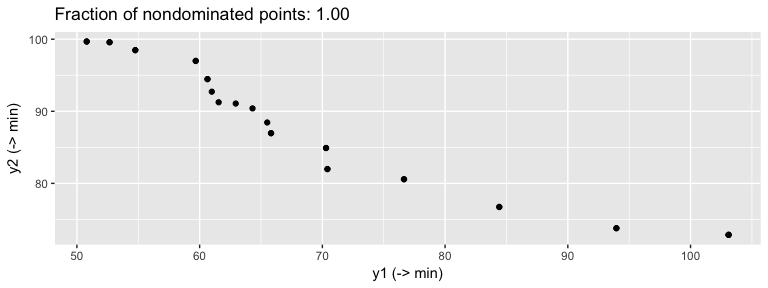Quickstart

The mcMST package for the statistical programming language R contains methods for benchmark instance generation of multi-objective graph problems and methods for solving the multi-criteria spanning tree problem (mcMST).

Generating a benchmark instance

Here we first generate a bi-criteria graph problem with n = 25 nodes. The first objective is the euclidean distance of node coordinates in [0, 10] x [0, 10] in the euclidean plane. The second objective follows a normal distribution with mean 5 and standard deviation 1.5. The instance generation process is modular and thus highly flexible (see the vignette on graph generation for details).

library(mcMST)
library(gridExtra)
set.seed(1) # reproducability
g = mcGP(lower = 0, upper = 10)
g = addCoordinates(g, n = 25, generator = coordUniform)
g = addWeights(g, method = "euclidean")
g = addWeights(g, method = "random", weight.fun = rnorm, mean = 5, sd = 1.5)
print(g)
#> MULTI-OBJECTIVE GRAPH PROBLEM
#> Number of nodes: 25
#> Weights per edge: 2 (distance,random)
plots = plot(g)
plots$nrow = 1 do.call(grid.arrange, plots)Plot of the bi-objective sample graph `g. Running an algorithm Next, we apply a (30 + 10) genetic algorithm based on the Pruefer-number encoding as proposed by Zhou & Gen to approximate the Pareto-front for max.iter = 500 generations. res = mcMSTEmoaZhou(g, mu = 30L, lambda = 10L, max.iter = 500L) head(res$pareto.front, n = 5)
#>          y1       y2
#> 1 103.10148 72.85682
#> 2  50.79960 99.67772
#> 3  50.79960 99.67772
#> 4 103.10148 72.85682
#> 5  84.41588 76.72809
ecr::plotFront(res\$pareto.front)Approximation of the Pareto-front of the benchmark graph instance.